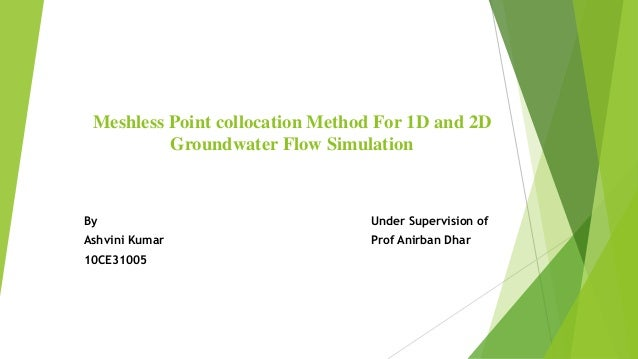Successfully reported this slideshow.Upcoming SlideShare
×

# Meshless Point collocation Method For 1D and 2D Groundwater Flow Simulation

902 views

Published on

This is my presentation of research on mesh less point collocation method for 1D and 2D groundwater flow simulation

Published in: Engineering
• Full Name
Comment goes here.

Are you sure you want to Yes No• Be the first to comment

### Meshless Point collocation Method For 1D and 2D Groundwater Flow Simulation

1. 1. Meshless Point collocation Method For 1D and 2D Groundwater Flow Simulation By Under Supervision of Ashvini Kumar Prof Anirban Dhar 10CE31005
2. 2. Introduction  Groundwater contamination and soil pollution have been recognized as critical environment problems therefore flow of groundwater must be analysed.  Analysing flow of ground water through analytical method or numerical methods like FEM and FDM or boundary element method is complex.  Meshfree method is a powerful numerical technique to obtain more accurate approximate solutions in a more convenient manner for complex systems.  This method is used to establish algebraic equation for the whole problem domain without the use of pre-defined mesh.  Different from FDM and FEM, Mfree method use a set of scatted nodes in the problem domain and boundaries of the domain.  In present project, Mfree model is developed for groundwater flow problems in 1D and 2D based on collocation techniques.
3. 3. Literature Review  Type of models use to study flow of groundwater can be classified in three categories  Sand Tank Models  Analog Model  Viscous Fluid Model  Electrical Models  Mathematical Models  Analytical Models  Numerical Models
4. 4. Problem Description In this project, a meshless method called as Polynomial Point collocation method (PPCM) with radial basis function has been developed for the groundwater flow simulation in porous media in one and two dimensions. The developed model has been applied for computing head distribution in a hypothetical confined aquifer having different boundary conditions and source, and sink terms. The developed model is tested with analytical and FEM solutions available in literature and found to be satisfactory.
5. 5. Development of PPCM Equation for analysis of flow of groundwater  Governing equation of flow of groundwater
6. 6. PPCM formulation for 1D transient Flow equation for confined aquifer  The transient of groundwater in homogeneous isotropic media in 1D can be written as Where, K is hydraulic conductivity (m/d) of the aquifer Sy is specific yield  To use Mfree method, the first step is to define the trial solution as where, is unknown head is the shape function at node I n is the total number of nodes in the support domain is given by and ℎ푖
7. 7. PPCM formulation for 1D transient Flow equation for confined aquifer  For MQ-RBF, q=0.5, therefore can be written as and therefore and Using earlier introduce equation, we get Arranging previous equation, we get (PPCM model for 1D flow of groundwater)
8. 8. PPCM formulation for 2D transient flow equation for confined aquifer  The transient of groundwater in homogeneous isotropic media in 2D can be written as  Again to use Mfree method, the first step is to define the trial solution as where,  Single and double derivatives of shape function w.r.t x and y can be written as  Putting these equation in first equation and arranging the terms, we get where, [K1] is global matrix of shape function [K2] is global matrix of double derivative of shape function w.r.t. x [K3] is global matrix of double derivative of shape function w.r.t y
9. 9. Model Development
10. 10. Support Domain and Collocation Point 1D Model 2D Model
11. 11. Verification of 1D Model
12. 12. Verification of 1D model
13. 13. Verification of 2D Model
14. 14. Verification of 2D Model Node No. t=0.2 days t=1day Analytical FEM % Error (analytical with FEM) PPCM %Error (Analytical with PPCM) FEM PPCM %Difference FEM with PPCM 29 97.013 96.993 0.02 97.0316 0.019 97.2316 97.2316 0.084 43 93.804 93.768 0.036 93.7606 0.046 94.0059 94.0059 0.047 57 90.095 90.051 0.044 90.0756 0.022 90.0245 90.0245 0.133 71 85.451 85.413 0.038 8 0.07 85.6105 85.6105 0.041 85 78.983 78.974 0.009 78.9544 0.024 78.8544 78.8544 0.272 99 67.953 67.762 0.191 67.8552 0.144 67.8212 67.8212 0.204
15. 15. Verification of 2D Model
16. 16. Case Study
17. 17. Case Study Properties ZONE I ZONE II ZONE III Transmissivity Tx (m2/d) 500 400 250 Transmissivity Ty (m2/d) 300 250 200 Porosity 0.2 0.25 0.15
18. 18. Case Study Node No. FEM PPCM % Difference 21 98.2 98.149 0.0129 34 97.1 96.927 0.0445 51 95.25 95.313 0.0165
19. 19. Case Study
20. 20. Thank You All